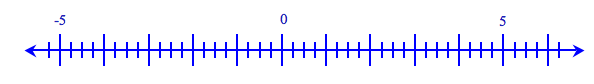### Home > CC1 > Chapter 6 > Lesson 6.2.1 > Problem6-73

6-73.

Draw a number line and place each of the following numbers in its appropriate place on the line: $-5\frac{1}{2}$, $−2$$4\frac{3}{4}$, $6$, $−1$, $\frac { 3 } { 2 }$, $−0.5$.

Making a number line like the one below (divided into fourths) will make the problem easier.